# if statement Python | if, if else, and if elif else in Python

The if, if-else, and if-elif-else statements are the most-used statements in the Python world. Since these provide for implementing conditions in a program, and based on those conditions, automatically or dynamically, the decisions can be taken by the program. Therefore, don't move forward without a complete understanding of these statements. This is similar to the fundamental building block of Python programming.

Therefore, I've provided all these three conditional statements in one single tutorial, along with their description, syntax, and easily understandable examples. This article deals with:

## if Statement

The "if" statement is used, where we've got to work with only one condition. That is, the program makes a decision based on only one condition, as specified by the if statement.

### if statement syntax

The syntax to use in an "if" statement in a Python program is:

```if expression:
statement(s)```

The expression indicates the conditional_expression code. The statement(s) of if get executed only if the conditional_expression code evaluates to be true. Otherwise, program flow does not go to the "if" body.

### if statement example

Let's take some examples to get a clearer understanding of the "if" statement. Here is a very basic example that uses an if statement:

```num = 10
print("Welcome to codescracker.com")
if num>0:
print("\"num\" holds a positive number.")
print("Exiting the program...")```

The output produced by the above program is: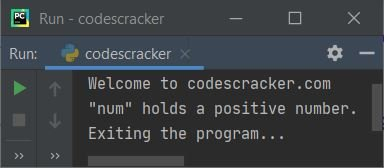Now let's modify the above program. That is, let's initialize the variable num with a value that is a negative number, like shown in the program given below, and re-run the program again to see its new output:

```num = -10
print("Welcome to codescracker.com")
if num>0:
print("\"num\" holds a positive number.")
print("Exiting the program...")```

Now this program produces the following output: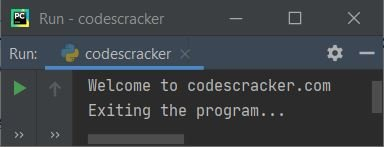As you can see from both example programs given above, In the first program, the condition "num>0" or "10>0" evaluates to be true; therefore, program flow goes inside the if's body and executes all the statements present inside its block of body. Since then, I've written only one statement; therefore, only that one statement gets executed, which prints ""num" holds a positive number" on output.

The rest of the two print statements, that is, the one written above the if and the second written below the if's body, doesn't care about if. That pair of statements always gets printed.

Now in the second program, since the value of num is -10 this time, the condition "num>0" or "-10>0" evaluates to be false. Therefore, program flow doesn't go inside the if's body. So the message "num" holds a positive number. doesn't get printed in the second program.

Let's take one more example of a Python if statement to provide you with a clue about how an if statement can also be used in a password-protected program:

```print("Welcome to codescracker.com")
pwd = input()
if pwd == "123@codescracker":
print("\nAccess Granted!")```

The snapshot given below shows the sample output produced by the above program after providing 123@codescracker as user input: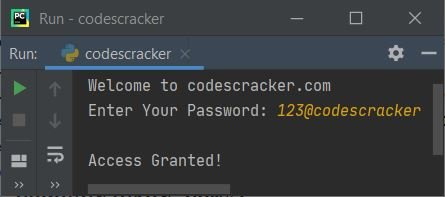That is, the user only gets access to some special section or feature if he or she enters the correct password. In the above program, I've only given you a hint about how an if statement can be used to protect some sections. Since this is just a demo program, I've directly compared the entered password with any random one, like 123@codescracker. But in a working application, generally, we fetched the user's password from the database and then checked it with his or her entered password.

### if statement without conditional expression

Note: The conditional expression used in the if statement is basically a boolean expression. A boolean expression is an expression that returns either true or false. After evaluating the boolean expression, we can get either true or false.

We can also replace the conditional expression in the if statement using a direct variable or object. In that case, what the variable or object's value is decides whether to enter it in the body or not.

These are the values listed, and they are all false:

• 0
• 0.0, or anything equivalent to 0
• None
• False
• '' (note the single empty quotation mark ' ')
• ""
• ()
• []
• {}

Except for the values listed above, all other values, such as 1, 24, "codescracker," and so on, are considered true.

As told above, if the variable's value is 0 or false, then it gets treated as false, and the program flow never goes to that if's body. Otherwise, all the variables' values (like 1, 2, -2, -4, etc.) including true get treated as true, therefore program flow goes inside the if's body. Let's take an example to get a complete understanding of it.

```num_one = 10
num_two = 0
num_three = True
num_four = False
num_five = -10

if num_one:
print("The value of \"num_one\" is", num_one)
if num_two:
print("The value of \"num_two\" is", num_two)
if num_three:
print("The value of \"num_three\" is", num_three)
if num_four:
print("The value of \"num_four\" is", num_four)
if num_five:
print("The value of \"num_five\" is", num_five)```

This program produces the output shown in the snapshot given below:### if statement in one line

If there is only one statement inside the body of if, we can write if in one line. Here is its syntax to use in one line:

`if expression: statement`

The example given below shows an if statement program with the whole if block created in one line:

```num = 10
if num>0: print("Positive")```

prints "Positive" on output. But a single-line if block cannot be used if there are multiple statements available in the if's body.

## if...else Statement

The if...else statement is basically the improved version of the if statement. That is, in the if statement of Python, we can only execute some sets of statements when the condition provided is true; otherwise, when the condition evaluates to be false, there is nothing to execute. For a change, if...else is designed to execute some sets of statements even if the condition is false.

That is, using "if...else" we'll have two bodies. One if statement and one else statement. The if's body statement(s) gets executed if the condition evaluates to be true; otherwise, if the condition evaluates to be false, then the else's body statement(s) gets executed.

### if...else Syntax

Let's have a look at the syntax of the "if...else" statement:

```if expression:
statement(s)
else:
statement(s)```

As you can see, we can execute statement(s) using else when the condition of if evaluates to false.

### if...else Example

In the programming world, we cannot understand things clearly and completely unless we implement them in a practical way. Therefore, I've provided you all the necessary programs that give more concentration and a complete understanding of the topic. Now in this if...else example section, I've taken the same example as provided for if, that is:

```num = 10
print("Welcome to codescracker.com")
if num>0:
print("\"num\" holds a positive number.")
else:
print("\"num\" holds zero or a negative number.")
print("Exiting the program...")```

The output produced by the above program is shown in the snapshot given below: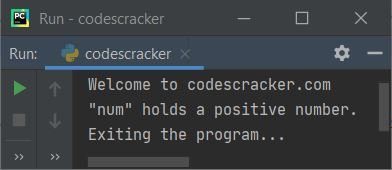Now let's change the above program as done in if's example:

```num = -10
print("Welcome to codescracker.com")
if num>0:
print("\"num\" holds a positive number.")
else:
print("\"num\" holds zero or a negative number.")
print("Exiting the program...")```

Now this time, since we've got an else block, which is the counterpart of if, we're able to execute some set of statements when the condition evaluates to be false. Here is its sample run: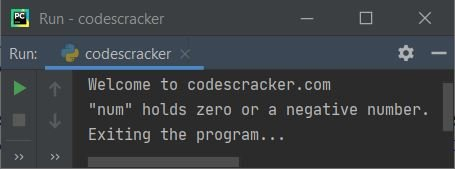As you can see, when the condition "num>0" or "-10>0" evaluates to false, then the program flow goes to the else's body and executes all the statements available in its body. Since in the above program, I've created only one statement inside the else's body, therefore, only that one statement gets executed, which prints "num," which holds zero or a negative number on the output console like shown in the snapshot given above.

Now let's recreate that password protected program using if-else logic that was created in the if section. I'm going to create the same program using if-else, it provides a good user experience (UX). Because we are using else, we are able to provide the message to the user that shows what happens if they enter a wrong password, as shown in the program given below:

```print("Welcome to codescracker.com")
pwd = input()
if pwd == "123@codescracker":
print("\nAccess Granted!")
else:

Here's a sample run with the user-supplied password codescracker. Since the password is 123@codescracker, not codescracker, therefore, you'll see the following output:### if...else without a conditional expression

Let's take one last example of an if-else statement. I hope this clears up any remaining uncertainty (if any) about if...else:

```num_one = 10
num_two = 0
num_three = True
num_four = False
num_five = -10

if num_one:
print("The value of \"num_one\" is", num_one)
else:
print("The value of \"num_on\" is", num_one)
if num_two:
print("The value of \"num_two\" is", num_two)
else:
print("The value of \"num_two\" is", num_two)
if num_three:
print("The value of \"num_three\" is", num_three)
else:
print("The value of \"num_three\" is", num_three)
if num_four:
print("The value of \"num_four\" is", num_four)
else:
print("The value of \"num_four\" is", num_four)
if num_five:
print("The value of \"num_five\" is", num_five)
else:
print("The value of \"num_five\" is", num_five)```

This program produces: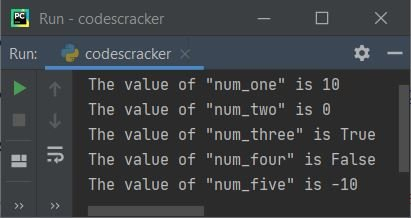As you can see, either the statement "if" or "else" gets executed, but not both in all five cases of the "if...else" block.

### if...else in a single line

This can be done with the help of a ternary operator. I've created a separate tutorial on the ternary operator or single-line if...else statement in Python.

## if...elif...else Statement

To use multiple conditions, we need an "if...elif...else" statement.

### if...elif...else Syntax

Here is the syntax used for the if...elif...else statement. We can create as many boolean expressions as we want.

```if expression_1:
statement(s)
elif expression_2:
statement(s)
elif expression_3:
statement(s)
.
.
.
elif expression_n:
statement(s)
else:
statement(s)```

The if...elif...else's syntax (as provided above) works in a way that:

• First, "expression_1" gets evaluated.
• If "expression_1" evaluates to "True," then program flow goes inside this "if"'s body and evaluates all of its "statement(s)."
• And if "expression_1" evaluates to "False,"  then program flow goes to the first "elif" and evaluates the "expression_2."
• If "expression_2" evaluates to "True,"  then program flow goes inside this "elif"'s body and evaluates all of its "statement(s)."
• And if "expression_2" evaluates to "False,"  then program flow goes to the second "elif" and evaluates the "expression_3."
• If "expression_3" evaluates to "True,"  then program flow goes inside this "elif"'s body and evaluates all of its "statement(s)."
• This process continues until the last expression, or "expression_n."
• The "statement(s)" of "else" gets executed only when the last expression (expression_n) evaluates to "False."
• While executing the expressions of the "if...elif...else" statement, if any expression evaluates to "True,"  then program flow goes to that body and executes all its statement(s). And the rest of the whole "if...elif...else" block gets skipped.
• That is, the process of evaluating all the expressions continues until we get "true." Otherwise, if all conditions evaluate to "False," the statement(s) in the "else" body are executed.

### if...elif...else Example

Let's take the first example of this tutorial again and get a better understanding of where and why we use if...elif...else statements:

```num = 10
print("Welcome to codescracker.com")
if num>0:
print("\"num\" holds a positive number.")
elif num==0:
print("\"num\" holds 0.")
else:
print("\"num\" holds a negative number.")
print("Exiting the program...")```

The output produced by the above program is exactly the same as the first program's output, as shown in the snapshot given below:Now, modify the program so that variable num has values of 1, 0, and -1 in one program, as shown in the program below:

```num = 1
print("\n--------When \"num = 1\"----------")
if num>0:
print("\"num\" holds a positive number.")
elif num==0:
print("\"num\" holds 0.")
else:
print("\"num\" holds a negative number.")

num = 0
print("\n--------When \"num = 0\"----------")
if num>0:
print("\"num\" holds a positive number.")
elif num==0:
print("\"num\" holds 0.")
else:
print("\"num\" holds a negative number.")

num = -1
print("\n--------When \"num = -1\"----------")
if num>0:
print("\"num\" holds a positive number.")
elif num==0:
print("\"num\" holds 0.")
else:
print("\"num\" holds a negative number.")```

The output produced by the above program is: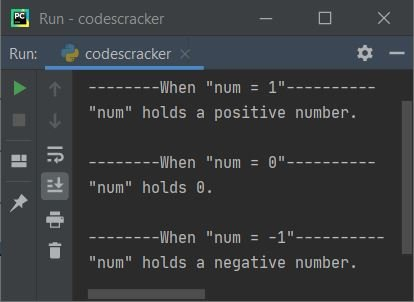## Nested if, if...else, if...elif...else statements

We can also nest one conditional statement inside another, like shown in the program given below:

```print("Enter any Number: ", end="")
num = int(input())

if num>0:
if num>10:
print("It is greater than 10.")
elif num>100:
if num>50:
print("It is greater than 50.")
else:
print("It is greater than 100.")
elif num>500:
print("It is greater than 500.")
elif num>1000000:
print("It is greater than 1000000.")
else:
print("It is less than 10.")
elif num==0:
print("It is 0.")
else:
if num>-10:
print("It is between -1 to -9.")
else:
print("It is less than -9.")```

Here is its sample run with user input 600: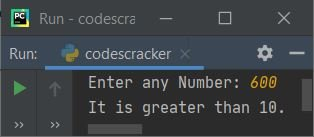Note: As you can see from the above program, since the first if's expression inside the first if is num>10 or 600>10, it evaluates to True. As a result, the rest of the expressions (of elifs) are not evaluated; instead, program flow goes inside this if's body and prints the message "It is greater than 10." And the rest of all conditional statements available in the same indentation get skipped.

For more programs on nested conditional statements, refer to Find the Largest of Three Numbers. There, I've created a program with nested if...elif...else that contains 18 conditional statements in a single program.

#### More Examples

These are the example programs that you may like to practice. All these programs contain if, if-else, or if-elif-else statements.

Python Online Test

« Previous Tutorial Next Tutorial »